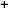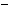Labware - MA35 Multivariable Calculus - Polar Coordinate Labs
 MA35 Labs » Polar Coordinate Labs Contents.1 Functions of Two Variables .1.1 Introduction .1.3 Slice Curves .1.5 Continuity Search
 Introduction TextCalculus is the study of functions.In the study of functions of one variable, we encounter domains and ranges of functions, function graphs, and properties of functions such as continuity. In multivariable calculus we will extend all of these notions to functions of two and eventually more variables. We will also deal with functions of one variable associated with functions of a single variable, namely slice curves and contour lines.A point is given in polar coordinates by an angle θ about the origin and a real number r, the distance from the origin.It is customary to write this as [r,θ]. A point can be converted from polar to Cartesian coordinates using the formulas $x = r \cos(\theta),$ $y = r \sin(\theta).$ Similarly, a point can be converted from Cartesian to polar coordinates using the formulas $r = \sqrt{x^2 + y^2},$ $\theta = \arctan (y/x) .$ExamplesZero FunctionsThe simplest function of all is the zero function, defined by f(r,θ) = 0 for all r,θ. This function can be defined for any domain, and the range will always always be the single point { 0 }.Constant FunctionsThe next simplest class of functions are the constant functions defined by f(r,θ) = k for all r,θ. A constant function can be defined for any domain, and the range will always always be the single point { k }.